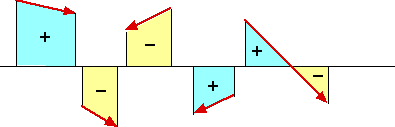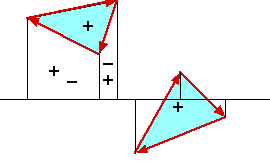### Area of Intersection of Polygons

This C program (Java too) computes the integral over the plane, of the product of the winding numbers of two polygons. If neither polygon is self intersecting and they have the same orientation, then this integral is the area of their intersection. Each polygon is provided to the program as an array of coordinate pairs, one for each vertex. Each pair of consecutive array elements describes a polygon edge; also the first and last array elements describe an edge. The routine is not confused by degenerate conditions such as coincident points or lines.
typedef struct{float x; float y;} point;
float inter(int na, point a[na], int nb, point b[nb]);

The arguments are two arrays of points, a and b, and their respective lengths, na and nb.

This program can be easily extended to compute moments of the intersection. Since the intersection of polygons may not be a polygon one cannot do the obvious thing of yielding a polygon as the intersection of two. One could introduce a polygon set abstraction and build the closed intersection operator for those. That, however, is more work than is necessary for the area.

#### Code Detail

The code uses nested procedures which is not legal ANSI C but the gcc compiler and several others support this extension to C.

An ANSI C version at github

It may seem strange to use a type such as hp in the program rather than double. It is necessary not for precision of the answer but to avoid common degenerate cases where points are nearly collinear. To avoid multitudinous degenerate cases, each of which is trivial but which are collectively overwhelming, I arrange to entirely avoid collinearity. The values of fudge and c&1 contrive to jiggle the points to avoid a vertex of one polygon lying on an edge of the other. The value of gamut is chosen to avoid overflow in routine area yet minimize the effect of the noise we add to the vertex coordinates. It has to do with there being about 232 values of an int. There must be no rounding of hp values. An untested assertion is thus that area should never return 0. If the signs of routine area were ever wrong then it would probably lead to intersections being missed and in turn gross errors in the output.

There are a few tests at the end that can be worked by hand. This intersects two regular triangles in many orientations to form a regular hexagon, and computes that area.

#### Mathematical Ideas

We associate an oriented area with each line segment that bounds the polygon. Here are some red segments and the associated areas. At each blue point the winding number is 1 and at each yellow point it is −1. It is 0 at white points.This is related to the elementary concept from integral calculus of area under the curve:
 ∫ y dx
over a range of x’s.
If we add winding numbers in the overlapping areas associated with each of the oriented sides of a polygon, they cancel except inside the polygon thus:The integral expression can be applied when the integral is taken around a closed curve, including negative dx’s. We have thus reduced an integral over the interior of a polygon to an integral over its boundary. The area is the unordered sum of a function of the oriented sides of the polygon. It is necessary for the logic of the program that this sum need not be ordered. This calculation works for collections of polygons as well, yielding the sum of the areas.

#### Comment on the Boundary of the Intersection

Let “∂x” denote the boundary of area x.
∂(x∩y) = x∩∂y ∪ ∂x∩y. Note the resemblance to the rule for the derivative of products. We reduce the integral over the intersection to an integral over the boundary of the intersection which, with this formula, requires finding the intersection of a polygon with the boundary of another.

Consider the integral below along the boundary of one simple polygon:
 ∫ w y dx
where w is the winding number of the other polygon. Add this to the integral swapping the two polygons and we have the integral along the boundary of the intersection of the two polygons, and thus the area of the intersection. For non simple polygons this is the integral over the plane of the product of their winding numbers.

Here we discuss the determination of the topology of the sides of the polygons. Here is a high level view of the algorithm with references to the code. See this for some fragmentary theory.

notes on non-convex polygon intersections

A 3D version.
This sounds like a general system.
an application